### Home > MC2 > Chapter Ch7 > Lesson 7.1.5 > Problem7-48

7-48.
1. There are 36 green gumballs, 18 red gumballs, 22 white gumballs, 30 purple gumballs, and 14 blue gumballs in the gumball machine. Find the probability for each of the following outcomes and write it as a percent. Assume that the gumball machine is always full and that gumballs are released from the machine randomly. Show work to support your answer. Homework Help ✎

1. Getting a purple gumball.

2. Getting either a purple or a green gumball.

3. Not getting a green gumball.

4. Getting either a purple or a white gumball.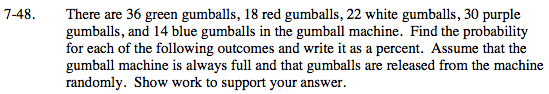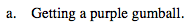The probability of getting a purple gumball is the number or purple gumballs divided by the total number of gumballs.

$\frac{30}{120} = 25$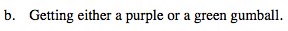The probability of getting either a purple or a green gumball is the sum of the probability of getting a purple gumball and the probability of getting a green gumball.

$\text{P(purple) + P(green)} = \frac{30}{120} + \frac{36}{120} = \frac{66}{120} = 55$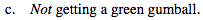What is the probability of getting a green gumball?

Since you can either get a green gumball or not get a green gumball, the probability of not getting a green gumball is 1 (or 100%) minus the probability of getting a green gumball.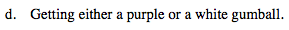What is the probability of getting a purple gumball?
A white gumball?

See (b).